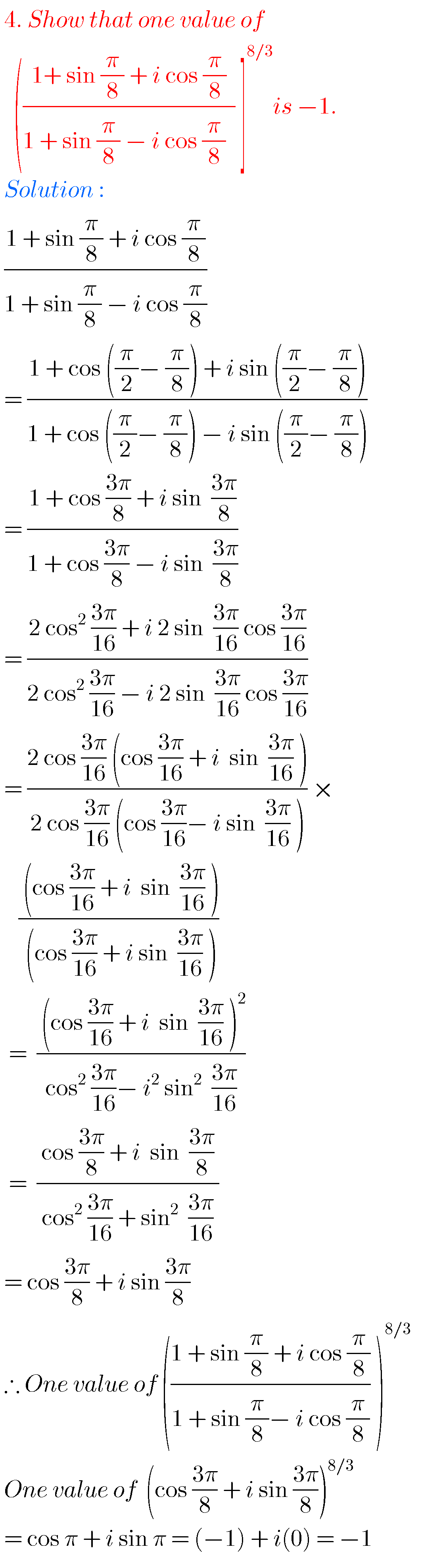## Intermediate maths solutions for De Moivre’s Theorem

Intermediate maths solutions for De Moivre’s Theorem Intermediate mathematics textbook chapter 2 exercises 2(a) and 2(b) solutions are given. These solutions are very easy to understand. First you study the textbook lesson. Then observe the example problems and solutions. Try them in your own way. Observe the solutions given below and try them in your …